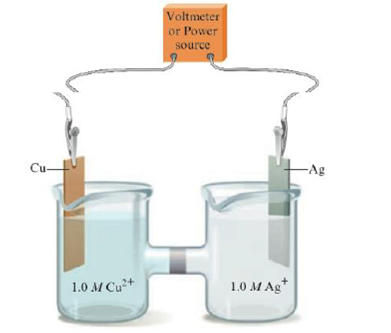# Consider the following electrochemical cell: a. If silver metal is a product of the reaction, is the cell a galvanic cell or electrolytic cell? Label the cathode and anode, and describe the direction of the electron flow. b. If copper metal is a product of the reaction, is the cell a galvanic cell or electrolytic cell? Label the cathode and anode, and describe the direction of the electron flow. c. If the above cell is a galvanic cell, determine the standard cell potential. d. If the above cell is an electrolytic cell, determine the minimum external potential that must be applied to cause the reaction to occur.### Chemistry: An Atoms First Approach

2nd Edition
Steven S. Zumdahl + 1 other
Publisher: Cengage Learning
ISBN: 9781305079243

#### Solutions

Chapter
Section### Chemistry: An Atoms First Approach

2nd Edition
Steven S. Zumdahl + 1 other
Publisher: Cengage Learning
ISBN: 9781305079243
Chapter 17, Problem 28Q
Textbook Problem
17 views

## Consider the following electrochemical cell:a. If silver metal is a product of the reaction, is the cell a galvanic cell or electrolytic cell? Label the cathode and anode, and describe the direction of the electron flow.b. If copper metal is a product of the reaction, is the cell a galvanic cell or electrolytic cell? Label the cathode and anode, and describe the direction of the electron flow.c. If the above cell is a galvanic cell, determine the standard cell potential.d. If the above cell is an electrolytic cell, determine the minimum external potential that must be applied to cause the reaction to occur.

(a)

Interpretation Introduction

Interpretation:

The electrochemical cell with copper and silver electrodes in contact with their electrolytic solutions is given. Various questions based on the given electrochemical cell are to be answered.

Concept introduction:

The galvanic cell converts chemical energy into electrical energy while the electrolytic cell converts electrical energy into chemical energy.

When the external cell potential becomes greater than the natural cell potential, it leads to the formation of an electrolytic cell.

To determine: The type of the cell formed when silver metal is the product of the reaction in the given electrochemical cell.

### Explanation of Solution

The given cell is galvanic cell if the silver metal is the product of the reaction.

The reactions taking placing over the anode is as follows,

CuCu2++2eE°anode=0.34V

The reaction taking place over the cathode is as follows,

2Ag++2e2AgE°cathode=0

(b)

Interpretation Introduction

Interpretation:

The electrochemical cell with copper and silver electrodes in contact with their electrolytic solutions is given. Various questions based on the given electrochemical cell are to be answered.

Concept introduction:

The galvanic cell converts chemical energy into electrical energy while the electrolytic cell converts electrical energy into chemical energy.

When the external cell potential becomes greater than the natural cell potential, it leads to the formation of an electrolytic cell.

To determine: The type of the cell formed if copper metal is the product of the reaction in the given electrochemical cell.

(c)

Interpretation Introduction

Interpretation:

The electrochemical cell with copper and silver electrodes in contact with their electrolytic solutions is given. Various questions based on the given electrochemical cell are to be answered.

Concept introduction:

The galvanic cell converts chemical energy into electrical energy while the electrolytic cell converts electrical energy into chemical energy.

When the external cell potential becomes greater than the natural cell potential, it leads to the formation of an electrolytic cell.

To determine: The standard cell potential if the given cell is a galvanic cell.

(d)

Interpretation Introduction

Interpretation:

The electrochemical cell with copper and silver electrodes in contact with their electrolytic solutions is given. Various questions based on the given electrochemical cell are to be answered.

Concept introduction:

The galvanic cell converts chemical energy into electrical energy while the electrolytic cell converts electrical energy into chemical energy.

When the external cell potential becomes greater than the natural cell potential, it leads to the formation of an electrolytic cell.

To determine: The minimum external potential that must be applied to cause the reactions to occur, if the given cell is an electrolytic cell.

### Still sussing out bartleby?

Check out a sample textbook solution.

See a sample solution

#### The Solution to Your Study Problems

Bartleby provides explanations to thousands of textbook problems written by our experts, many with advanced degrees!

Get Started

Find more solutions based on key concepts
The freezing point of water is _________ K.

Introductory Chemistry: A Foundation

The DRI are for all people, regardless of their medical history. T F

Nutrition: Concepts and Controversies - Standalone book (MindTap Course List)

A cars speedometer reads instantaneous ___. (2.2)

An Introduction to Physical Science

How long does it take light to cross the diameter of our Milky Way Galaxy?

Horizons: Exploring the Universe (MindTap Course List)

What impact has recombinant DNA technology had on genetics and society?

Human Heredity: Principles and Issues (MindTap Course List)

Express 45 milliliters as fluid ounces.

Introductory Chemistry: An Active Learning Approach

Describe evidence of geological activity on Triton.

Foundations of Astronomy (MindTap Course List)

How might unnatural sounds affect the behavior of marine organisms or the health of communities?

Oceanography: An Invitation To Marine Science, Loose-leaf Versin

A truck loaded with sand accelerates along a highway. The driving force on the truck remains constant. What hap...

Physics for Scientists and Engineers, Technology Update (No access codes included)